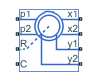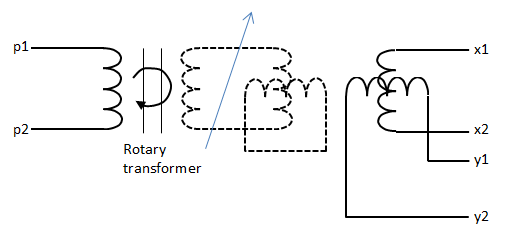# Resolver

Rotary transformer that measures angle of rotation

• Library:
• Simscape / Electrical / Sensors & Transducers

•## Description

The Resolver block models a generic resolver, which measures the electrical phase angle of a signal through electromagnetic coupling. The resolver consists of a rotary transformer that couples an AC voltage applied to the primary winding to two secondary windings. These secondary windings are physically oriented at 90 degrees to each other. As the rotor angle changes, the relative coupling between the primary and the two secondary windings varies. In the Resolver block model, the first secondary winding is oriented such that peak coupling occurs when the rotor is at zero degrees, and therefore the second secondary winding has minimum coupling when the rotor is at zero degrees.Without loss of generality, it is assumed that the transformer between primary and rotor circuit is ideal with a ratio of 1:1. This results in the rotor current and voltage being equivalent to the primary current and voltage.

You have two options for defining the block equations:

• Omit the dynamics by neglecting the transformer inductive terms. This model is only valid if the sensor is driven by a sine wave because any DC component on the primary side will pass to the output side.

• Include the inductive terms, thereby capturing voltage amplitude loss and phase differences. This model is valid for any input waveform. Within this option, you can either specify the inductances and the peak coupling coefficient directly, or specify the transformation ratio and measured impedances, in which case the block uses these values to determine the inductive terms.

### Equations when Omitting Dynamics

The equations are based on the superposition of two ideal transformers, both with coupling coefficients that depend on rotor angle. The two ideal transformers have a common primary winding. See the Simscape™ Ideal Transformer block reference page for more information on modeling ideal transformers. The equations are:

Kx = R cos( N Θ )

Ky = R sin( N Θ )

vx = Kx v p

vy = Ky v p

ip = – Kx ixKy iy

where:

• vp and ip are the rotor (or equivalently primary) voltage and current, respectively.

• vx and ix are the first secondary voltage and current, respectively.

• vy and iy are the second secondary voltage and current, respectively.

• Kx is the coupling coefficient for the first secondary winding.

• Ky is the coupling coefficient for the second secondary winding.

• R is the transformation ratio.

• N is the number of pole pairs.

• Θ is the rotor angle.

### Equations when Including Dynamics

The equations are based on the superposition of two mutual inductors, both with coupling coefficients that depend on rotor angle. The two mutual inductors have a common primary winding. See the Simscape Mutual Inductor block reference page for more information on modeling mutual inductors. The equations are:

`${v}_{p}={R}_{p}{i}_{p}+{L}_{p}\frac{d{i}_{p}}{dt}+\sqrt{{L}_{p}{L}_{s}}k\left(\mathrm{cos}\left(N\theta \right)\frac{d{i}_{x}}{dt}+\mathrm{sin}\left(N\theta \right)\frac{d{i}_{y}}{dt}\right)$`
`${v}_{x}={R}_{s}{i}_{x}+{L}_{s}\frac{d{i}_{x}}{dt}+\sqrt{{L}_{p}{L}_{s}}k\mathrm{cos}\left(N\theta \right)\frac{d{i}_{p}}{dt}$`
`${v}_{y}={R}_{s}{i}_{y}+{L}_{s}\frac{d{i}_{y}}{dt}+\sqrt{{L}_{p}{L}_{s}}k\mathrm{sin}\left(N\theta \right)\frac{d{i}_{p}}{dt}$`

where:

• vp and ip are the rotor (or equivalently primary) voltage and current, respectively.

• vx and ix are the first secondary voltage and current, respectively.

• vy and iy are the second secondary voltage and current, respectively.

• Rp is the rotor (or primary) resistance.

• Lp is the rotor (or primary) inductance.

• Rs is the stator (or secondary) resistance.

• Ls is the stator (or secondary) inductance.

• N is the number of pole pairs.

• k is the coefficient of coupling.

• Θ is the rotor angle.

It is assumed that coupling between the two secondary windings is zero.

Datasheets typically do not quote the coefficient of coupling and inductance parameters, but instead give the transformation ratio R and measured impedances. If you select ```Specify transformation ratio and measured impedances``` for the Parameterization parameter, then the values you provide are used to determine values for the equation coefficients, as defined above.

### Variables

To set the priority and initial target values for the block variables prior to simulation, use the Initial Targets section in the block dialog box or Property Inspector. For more information, see Set Priority and Initial Target for Block Variables.

Nominal values provide a way to specify the expected magnitude of a variable in a model. Using system scaling based on nominal values increases the simulation robustness. Nominal values can come from different sources, one of which is the Nominal Values section in the block dialog box or Property Inspector. For more information, see System Scaling by Nominal Values.

## Assumptions and Limitations

• The resolver draws no torque between the mechanical rotational ports R and C.

• The transformer between primary and rotor circuit is ideal with a ratio of 1:1.

• The coupling between the two secondary windings is zero.

## Ports

### Conserving

expand all

Electrical conserving port associated with the positive terminal of the primary winding.

Electrical conserving port associated with the negative terminal of the primary winding.

Mechanical rotational conserving port connected to the rotor.

Mechanical rotational conserving port connected to the resolver case.

Electrical conserving port associated with the positive terminal of secondary winding x.

Electrical conserving port associated with the negative terminal of secondary winding x.

Electrical conserving port associated with the positive terminal of secondary winding y.

Electrical conserving port associated with the negative terminal of secondary winding y.

## Parameters

expand all

Select one of the following methods for block parameterization:

• ```Specify transformation ratio and omit dynamics``` — Provide values for transformation ratio, number of pole pairs, and initial rotor angle only. This model neglects the transformer inductive terms, and is only valid if the sensor is driven by a sine wave. The equations are based on the superposition of two ideal transformers, both with coupling coefficients that depend on rotor angle. For more information, see Equations when Omitting Dynamics.

• ```Specify transformation ratio and measured impedances``` — Provide additional values to determine the transformer inductive terms, to model the voltage amplitude loss and phase differences. This model is valid for any input waveform. The equations are based on the superposition of two mutual inductors, both with coupling coefficients that depend on rotor angle. For more information, see Equations when Including Dynamics.

• ```Specify equation parameters directly``` — Model the dynamics, but provide values for rotor and stator inductances and the peak coefficient of coupling, instead of transformation ratio and measured impedances. For more information, see Equations when Including Dynamics. This model is valid for any input waveform.

Ratio between the peak output voltage and the peak input voltage assuming negligible secondary voltage drop due to resistance and inductance.

#### Dependencies

To enable this parameter, set the Parameterization parameter to ```Specify transformation ratio and omit dynamics``` or ```Specify transformation ratio and measured impedances```. If you select ```Specify transformation ratio and measured impedances``` for the Parameterization parameter, then the transformation ratio takes the voltage drop due to primary winding resistance into account.

Rotor ohmic resistance. This resistance is also referred to as the primary resistance.

#### Dependencies

To enable this parameter, set the Parameterization parameter to ```Specify transformation ratio and measured impedances``` or ```Specify equation parameters directly```.

Stator ohmic resistance. This resistance is also referred to as the secondary resistance. It is assumed that both secondaries have the same resistance.

#### Dependencies

To enable this parameter, set the Parameterization parameter to ```Specify transformation ratio and measured impedances``` or ```Specify equation parameters directly```.

Rotor reactance when the secondary windings are open-circuit. This reactance is also referred to as the primary reactance.

#### Dependencies

To enable this parameter, set the Parameterization parameter to ```Specify transformation ratio and measured impedances```.

Stator reactance when the primary winding is open-circuit. This reactance is also referred to as the secondary reactance.

#### Dependencies

To enable this parameter, set the Parameterization parameter to ```Specify transformation ratio and measured impedances```.

Frequency of the sinusoidal source used when measuring the reactances.

#### Dependencies

To enable this parameter, set the Parameterization parameter to ```Specify transformation ratio and measured impedances```.

Rotor or primary inductance, Lp.

#### Dependencies

To enable this parameter, set the Parameterization parameter to ```Specify equation parameters directly```.

Stator or secondary inductance, Ls.

#### Dependencies

To enable this parameter, set the Parameterization parameter to ```Specify equation parameters directly```.

Peak coefficient of coupling between the primary and secondary windings.

#### Dependencies

To enable this parameter, set the Parameterization parameter to ```Specify equation parameters directly```.

Number of pole pairs on the rotor.

## Version History

Introduced in R2017b